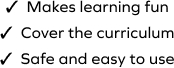# Determine the Missing Number in Subtraction

SplashLearn - The complete PreK - Grade 5 Math & ELA Learning Program Built for Your Child
Home > Determine the Missing Number in Subtraction
The game requires students to work with a set of problems on subtraction and use their conceptual understanding to find the missing numbers in math sentences. This set of problems deals with subtraction facts of 7. Students will get opportunities to work with different sets along the way. Students will use their understanding of subtraction to drag the correct responses.